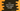# C++ print inverted right-angled triangle# C++ inverted right-angled triangle :

We can print one inverted right-angled triangle using two loops. In this C++ tutorial, we will use two for loops. The program will take the height of the triangle as input from the user and print out the triangle.

The program will also take the character to print the triangle as user input. You can use different characters to print the triangle.

## Example program :

``````#include <iostream>
using namespace std;

void printInvertedTriangle(int size, char c)
{
// 4
for (int i = 1; i <= size; i++) { for (int j = size; j >= i; j--)
{
cout << c << " ";
}
// 5
cout << "\n";
}
}

int main()
{
// 1
int size;
char c;

// 2
cout << "Enter the size : " << endl; cin >> size;

cout << "Enter the character : " << endl; cin >> c;

// 3
printInvertedTriangle(size, c);

return 0;
}``````

### Explanation :

The commented numbers in the above program denote the step numbers below :

1. Create two variables size and c to store the size of the triangle and the character to print the triangle.
2. Ask the user to enter the size of the triangle. Read it and store it in size. Similarly, read the character to print the triangle and store it in c.
3. Call the printInvertedTriangle method to print the triangle.
4. Inside printInvertedTriangle, we are using two for loops. The outer loop denotes the current line. It runs for size amount of time. The inner will run from j = size to j = i. Inside this inner loop, print the value of the character c that is passed to printInvertedTriangle.
5. Once the inner loop completes, move to the next line by printing a newline ‘\n’.

### Sample Output :

``````Enter the size :
5
Enter the character :
*
* * * * *
* * * *
* * *
* *
*``````